+450
4.5 to 1Jonathan Taylor
11.5% implied probability

+650
6.5 to 1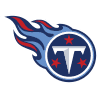Derrick Henry
8.4% implied probability

+1000
10 to 1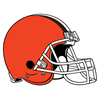Nick Chubb
5.7% implied probability

+1000
10 to 1Dalvin Cook
5.7% implied probability

+1400
14 to 1Najee Harris
4.2% implied probability

+1400
14 to 1Joe Mixon
4.2% implied probability

+2000
20 to 1Javonte Williams
3.0% implied probability

+2500
25 to 1Elijah Mitchell
2.4% implied probability

+3000
30 to 1Cam Akers
2.0% implied probability

+3000
30 to 1Rashaad Penny
2.0% implied probability

+3000
30 to 1Ezekiel Elliott
2.0% implied probability

+3500
35 to 1J.K. Dobbins
1.8% implied probability

+3500
35 to 1Damien Harris
1.8% implied probability

+3500
35 to 1Austin Ekeler
1.8% implied probability

+3500
35 to 1Antonio Gibson
1.8% implied probability

+3500
35 to 1Christian McCaffrey
1.8% implied probability

+4000
40 to 1Lamar Jackson
1.5% implied probability

+4000
40 to 1Josh Jacobs
1.5% implied probability

+4000
40 to 1Leonard Fournette
1.5% implied probability

+4000
40 to 1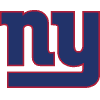Saquon Barkley
1.5% implied probability

+5000
50 to 1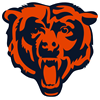David Montgomery
1.2% implied probability

+5000
50 to 1Miles Sanders
1.2% implied probability

+5000
50 to 1Devin Singletary
1.2% implied probability

+5000
50 to 1Breece Hall
1.2% implied probability

+5000
50 to 1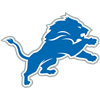D'Andre Swift
1.2% implied probability

+6000
60 to 1Kenneth Walker
1.0% implied probability

+6000
60 to 1James Conner
1.0% implied probability

+6000
60 to 1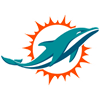Chase Edmonds
1.0% implied probability

+6000
60 to 1Ronald Jones
1.0% implied probability

+6000
60 to 1Aaron Jones
1.0% implied probability

+6000
60 to 1Alvin Kamara
1.0% implied probability

+8000
80 to 1AJ Dillon
0.8% implied probability

+8000
80 to 1Melvin Gordon
0.8% implied probability

+8000
80 to 1Tony Pollard
0.8% implied probability

+10000
100 to 1Travis Etienne
0.6% implied probability

+10000
100 to 1Clyde Edwards-Helaire
0.6% implied probability

+10000
100 to 1Jalen Hurts
0.6% implied probability

+10000
100 to 1Sony Michel
0.6% implied probability

+10000
100 to 1James Robinson
0.6% implied probability

+10000
100 to 1Rhamondre Stevenson
0.6% implied probability

+10000
100 to 1Mark Ingram
0.6% implied probability

+10000
100 to 1Michael Carter
0.6% implied probability

+10000
100 to 1Darrell Henderson
0.6% implied probability

+10000
100 to 1James Cook
0.6% implied probability

+10000
100 to 1Raheem Mostert
0.6% implied probability

+13000
130 to 1Isaiah Spiller
0.5% implied probability

+13000
130 to 1Khalil Herbert
0.5% implied probability

+15000
150 to 1Kareem Hunt
0.4% implied probability

+15000
150 to 1Phillip Lindsay
0.4% implied probability

+15000
150 to 1Trey Sermon
0.4% implied probability

+15000
150 to 1Josh Allen
0.4% implied probability

+15000
150 to 1Gus Edwards
0.4% implied probability

+15000
150 to 1Kyler Murray
0.4% implied probability

+20000
200 to 1Jamaal Williams
0.3% implied probability

+20000
200 to 1Mike Davis
0.3% implied probability

+20000
200 to 1Cordarrelle Patterson
0.3% implied probability

+20000
200 to 1Jerick McKinnon
0.3% implied probability

+20000
200 to 1Latavius Murray
0.3% implied probability

+20000
200 to 1Deebo Samuel
0.3% implied probability

+20000
200 to 1Darrel Williams
0.3% implied probability

+20000
200 to 1David Johnson
0.3% implied probability

+20000
200 to 1Devonta Freeman
0.3% implied probability

+20000
200 to 1Justin Fields
0.3% implied probability

+20000
200 to 1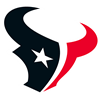Marlon Mack
0.3% implied probability

+20000
200 to 1Boston Scott
0.3% implied probability

+20000
200 to 1Jeff Wilson
0.3% implied probability

+20000
200 to 1Kenyan Drake
0.3% implied probability

+20000
200 to 1D'Onta Foreman
0.3% implied probability

+20000
200 to 1Alexander Mattison
0.3% implied probability

+20000
200 to 1D'Ernest Johnson
0.3% implied probability

+20000
200 to 1Chuba Hubbard
0.3% implied probability

+20000
200 to 1Nyheim Hines
0.3% implied probability

+20000
200 to 1Rex Burkhead
0.3% implied probability

+25000
250 to 1Justin Jackson
0.3% implied probability

+25000
250 to 1Ty Johnson
0.3% implied probability

+25000
250 to 1J.D. McKissic
0.3% implied probability

+25000
250 to 1Kenneth Gainwell
0.3% implied probability

+25000
250 to 1Zack Moss
0.3% implied probability

+25000
250 to 1Myles Gaskin
0.3% implied probability

+25000
250 to 1Samaje Perine
0.3% implied probability

+25000
250 to 1Tevin Coleman
0.3% implied probability

+25000
250 to 1James White
0.3% implied probability

+25000
250 to 1Dameon Pierce
0.3% implied probability

No changes have been recorded yet.